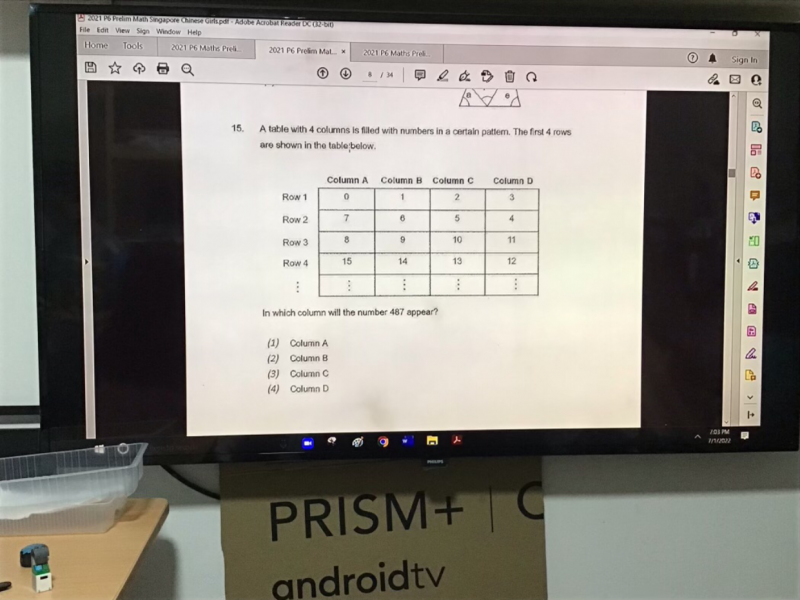# QuestionPls help to sovle thx

There are 4 columns => 4 sequences

In each sequence, we only need to look at the odd number sequence, because 487 is an odd number.

7,15,….   start = 7  diff = 8

1,9… start = 1,  diff = 8

5,13…. start = 5 diff = 8

3,11….start = 3, diff = 8

nth term = first term + (n – 1) x difference

7+(n-1)8 = 487

8n – 8 = 480

8n = 488 =>  n = 61 (whole number)   The rest are not whole numbers.

Hence the answer is (1) Column A